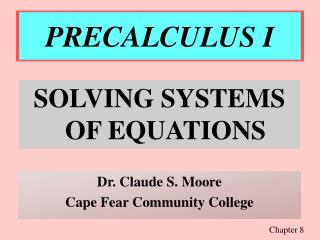Download PresentationPRECALCULUS I

# PRECALCULUS I - PowerPoint PPT PresentationDownload Presentation## PRECALCULUS I

- - - - - - - - - - - - - - - - - - - - - - - - - - - E N D - - - - - - - - - - - - - - - - - - - - - - - - - - -
##### Presentation Transcript

1. PRECALCULUS I SOLVING SYSTEMS OF EQUATIONS Dr. Claude S. Moore Cape Fear Community College Chapter 8

2. PRECALCULUS I TWO-VARIABLE LINEAR SYSTEMS 672

3. GRAPHICAL METHOD 1. Graph each equation on the same coordinate (x-y) plane. 2. Find the point(s) of intersection, if any exist. 3. Check the solution(s) in each of the original equations. 672

4. INTERPRETING GRAPHS of two linear equations in two variables: Number of solutions 1. Exactly one consistent; independent 2. Infinitely many consistent; dependent 3. No solution inconsistent Graph interpretations 1. Intersect in one point 2. Lines are identical 3. Lines are parallel 673

5. GRAPHICAL: EXAMPLE 1 Graph and solve (1) 8x + 9y = 42(2) 6x - y = 16 (1) (0,42/9); (42/8,0) (2) (0,-16); (16/6,0) 1 2 The solution is (3,2) which checks in both equations. 673

6. ELIMINATION METHOD 1. Get coefficients of x (or y) to be opposites of each other. 2. Add equations to eliminate variable. 3. Back-substitute into either equation. 4. Check your solution in both of the original equations. 674

7. ELIMINATION: EXAMPLE 2 Solve by elimination: (1) 5u + 6v = 32 (2) 3u + 5v = 22 -3(1) -15u - 18v = - 96 5(2) 15u + 25v = 110 (3) 7v = 14 v = 2 In equation (2), substitute v = 2: 3u + 5(2) = 22 3u + 10 = 22 3u = 12 u = 4Solution is (4,2). 675

8. PRECALCULUS I MULTIVARIABLE LINEAR SYSTEMS 688

9. ROW-ECHELON FORM The Gausian elimination process was named for Carl Friedrich Gauss (1777-1855) a German mathematician who developed the row-echelon form . 688

10. ROW-ECHELON FORM x - 2y + 3z = 9 -x + 3y = - 4 2x - 5y + 5z = 17 This system is in its original form. x - 2y + 3z = 9 y + 3z = 5 z = 2 This equivalent system is in Row-Echelon form. ?

11. ROW OPERATIONS: EQUIVALENT SYSTEMS 1. Interchange two equations. 2. Multiply one equation by non-zero constant. 3. Add a non-zero multiple of one equation to a non-zero multiple of another equation. 688

12. INCONSISTENT SYSTEM Solve the system of equations: E1 3x - 2y - 6z = -4 E2 -3x + 2y + 6z = 1 E3 x - y - 5z = -3 e1 x - y - 5z = -3 -3e1+e2 y + 9z = 5 3e1+e3 - 1y - 9z = -8 e1 x - y - 5z = -3 e2 3x - 2y - 6z = -4 e3 -3x + 2y + 6z = 1 e1 x - y - 5z = -3 e2 y + 9z = 5 e2+e3 0z = -3 Since 0 = -3 is never true, there is no solution. 691

13. INFINITE SOLUTION Solve the system of equations: E1 x + 2y - 7z = -4 E2 2x + y + z = 13 E3 3x + 9y -36z = -33 e1 x + 2y - 7z = -4 e2-2e1 -3y + 15z = 21 e3-3e1 3y - 15z = -21 e1 x + 2y - 7z = -4 (-1/3)e2 y - 5z = -7 e3+e1 0z = 0 Since 0z = 0 is always true, the solution (-3a+10, 5a-7, a) is infinite. 691

14. NON-SQUARE SYSTEM Solve the system of equations: E1 x - 3y + 2z = 18 E2 5x - 13y + 12z = 80 e1 x - 3y + 2z = 18 (1/2)e2 y + z = -5 So y = -z - 5. Let z =a. Then y = -a - 5. e1 x - 3y + 2z = 18 -5e1+e2 2y + 2z = -10 Substitute z = a and y = -a -5 into equation 1 and get the solution (-5a + 3, -a - 5, a). ?

15. PRECALCULUS I TWO-VARIABLE NON-LINEAR SYSTEMS 672

16. SUBSTITUTION METHOD 1. Solve one equation for one variable. 2. Substitute into other equation. 3. Solve equation from Step 2. 4. Back-substitute into Step 1. 5. Check the solution in each equation. Section 9-4

17. SUBSTITUTION: EXAMPLE 1 Solve x + y = 0 and x3 - 5x - y = 0. Substitute y = -x to get x3 - 5x - (-x) = 0 x3 - 5x + x = 0 or x3 - 4x = 0 x(x2 - 4) = 0 or x(x + 2)(x - 2) = 0 Thus x = 0, x = -2, and x = 2 giving the solutions (-2,2), (0,0), and (2,-2). Section 9-4

18. GRAPHICAL METHOD 1. Graph each equation on the same coordinate (x-y) plane. 2. Find the point(s) of intersection, if any exist. 3. Check the solution(s) in each of the original equations. Section 9-4

19. GRAPHICAL: EXAMPLE 2 Graph and solve y = ex & x - y = -1 x - y = -1 yields y = x + 1. Solution is (0,1) which checks in both equations: 1 = e0 and 0 - 1 = -1. Section 9-4

20. GRAPHICAL: EXAMPLE 3 Graph and solve (1) 2x - y = 1(2) x2 + y = 2 (1) y = 2x - 1(2) y = -x2 + 2. Solutions are (-3,-7) & (1,1) which check in both equations. Section 9-4